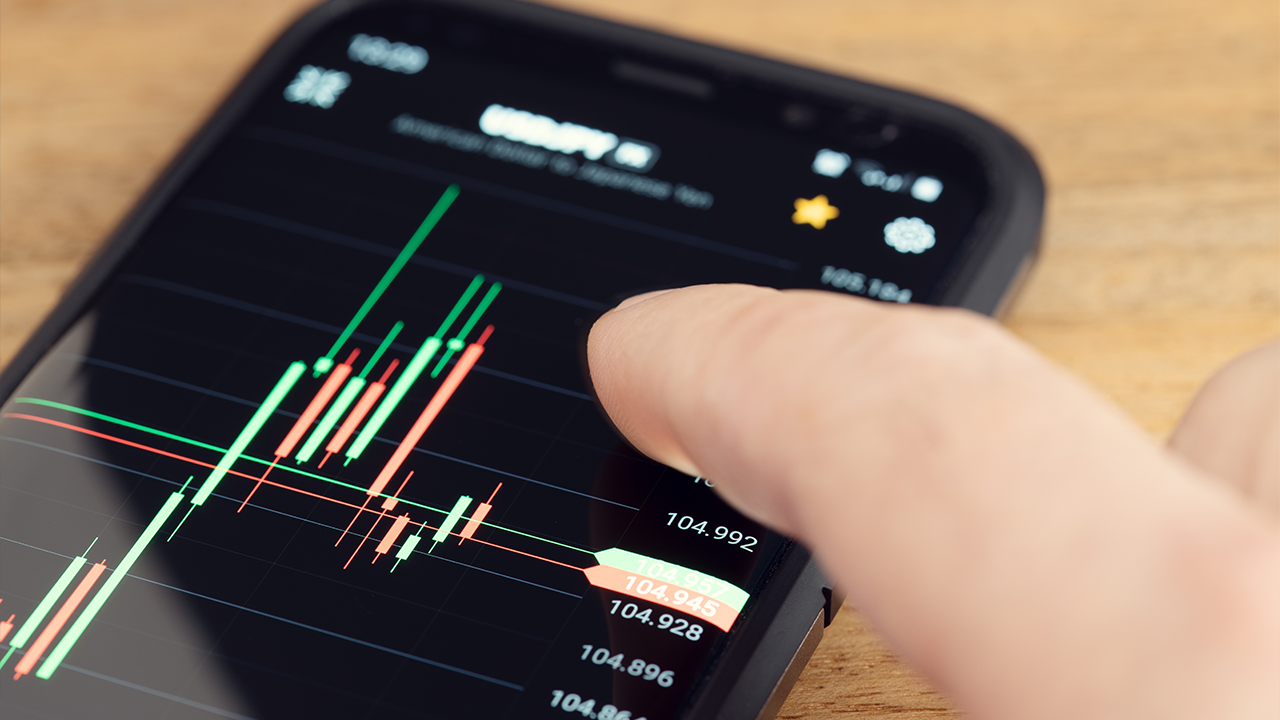FREE

May 22, 2021

# Trading for Beginners: What is a Pip?The chances are, if you’ve started learning about trading, you’ve probably heard the term ‘pip’. It’s a pretty common term in forex trading, but what is a pip?

A pip is simply how we measure the smallest price movement that can happen within a currency pair.

In the majority of pairs one pip will be equal to the fourth decimal place.

Let’s take EUR/USD as an example and assume the price is currently 1.1500. If the price moves up to 1.1501 that movement is one pip. Easy, right?

However, some currency pairs do differ slightly. The most common example of a major currency that differs to that is the Japanese yen.

In currency pairs where the yen is the quote currency, a pip would be the second decimal place. So if the price of USD/JPY moves from 100.100 to 100.110, that would be a one pip move.

You may find that some brokers will add another decimal point to the end, which is known as a point or a fractional pip, but you don’t need to worry about that for now.

What’s the point of understanding this then?

Well, pips actually have a number of practical uses for us besides just seeing how far the price has moved.

On the risk management side, you can use pips to measure the distance between a potential entry price and your stop loss. This can then be used to calculate an appropriate position size.

Once you have calculated an appropriate position size, you will now know how much money a one pip move is worth to you. So if the price moves 10 pips in your favour, you’ll know how much profit that is. It will be the number of pips, in this case 10, multiplied by the amount of money one pip is worth to you.

Likewise, if the price moves against you, you’ll be able to calculate how big the loss is. So if you know your stop loss is 10 pips away, you will know exactly how much that loss is in monetary terms.

Measuring pips is usually pretty straightforward with most trading platforms, so don’t worry if maths isn’t your strong point. There will typically be a crosshair which you can drag across the move you’re wanting to measure and this will show you how many pips it was.

If you want to build on this knowledge and learn more about trading, I’d recommend getting started with our free training to begin learning the Duomo Method.

go  top